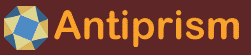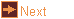# EXTRA: poly_weave - weave based on a polyhedron

 Usage    |    Examples    |    Notes

## Usage

```

Usage: poly_weave [options] [input_file]

Read a file in OFF format and make a weave following a specified pattern.
The polyhedron faces are divided by a 'meta' operation into triangles
each having vertices which are a vertex V, edge centre E and face centre F.
A start point is positioned on one of these triangles, the next point is
found by using the pattern to step between triangles, leading to a circuit.
Intermediate points may be added along the steps to help with the weave.
If input_file is not given the program reads from standard input.

Options
-h,--help this help message (run 'off_util -H help' for general help)
--version version information
-p <pat>  weave pattern (default 'FEV'), a series of one or more paths.
A path is:
C,L - path of intermediate points is curve (default),line
(affects following paths, set as first char of pattern)
Barycentric coords V,E,F of initial point (default 0,1,0)
Optional intermediate points on the path - ':' followed
by 0 to 3 coordinates for 'up' (def: 0), 'side' (def: 0),
'along' (def: equal spacing)
Series of characters to step between triangles
-     - stay on the same triangle
V,E,F - step two triangles rotating about V,E,F
R     - R reverse direction of following rotations
v,e,f - step over side opposite V,E,F
t followed by l,r,b (def: tl), start circuits from 'left',
'right', both triangles
-f <ht>   lift the face centres by this height
-o <file> write output to file (default: write to standard output)

```

## Examples

An interwoven set of six pentagons

```poly_weave -o woven_pents.off icosahedron
```

An interwoven set of six pentagons. To show the circuits of the weave, colour the edges by face colour and hide the faces
```poly_weave icosahedron | off_color -f U -e F | antiview -v 0.1 -x f
```

## Notes

The poly_weave program was a starting point for creating the wythoff tiling program.